International
Tables for
Crystallography
Volume D
Physical properties of crystals
Edited by A. Authier

International Tables for Crystallography (2006). Vol. D, ch. 1.1, pp. 26-27

## Section 1.1.4.10.5. Reduction of the number of independent components of fourth-rank polar tensors due to the symmetry of the strain and stress tensors

A. Authiera*

aInstitut de Minéralogie et de la Physique des Milieux Condensés, Bâtiment 7, 140 rue de Lourmel, 75015 Paris, France
Correspondence e-mail: aauthier@wanadoo.fr

#### 1.1.4.10.5. Reduction of the number of independent components of fourth-rank polar tensors due to the symmetry of the strain and stress tensors

| top | pdf |

Let us consider five examples of fourth-rank tensors:

 (i) Elastic compliances,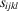, relating the resulting strain tensorto an applied stress(see Section 1.3.3.2):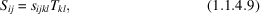where the compliancesare the components of a tensor of rank 4. (ii) Elastic stiffnesses,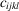(see Section 1.3.3.2):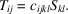(iii) Piezo-optic coefficients,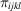, relating the variation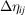of the dielectric impermeability to an applied stress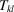(photoelastic effect – see Section 1.6.7):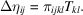(iv) Elasto-optic coefficients,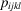, relating the variationof the dielectric impermeability to the strain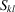: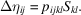(v) Electrostriction coefficients,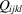, which appear in equation (1.1.4.4):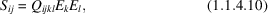where only the second-order terms are considered.

In each of the equations from (1.1.4.9)to (1.1.4.10), the contracted product of a fourth-rank tensor by a symmetric second-rank tensor is equal to a symmetric second-rank tensor. As in the case of the third-rank tensors, this results in a reduction of the number of independent components, but because of the properties of the strain Voigt matrix, and because two of the tensors are endowed with intrinsic symmetry (the elastic tensors), the reduction is different for each of the five tensors. The above relations can be written in matrix form:where the second-rank tensors are represented by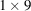column matrices, which can each be subdivided into three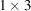sub­matrices and thematrix associated with the fourth-rank tensors is subdivided into ninesubmatrices, as shown in Section 1.1.4.9.1. The symmetry of the second-rank tensors means that submatrices 2 and 3 which are associated with them are equal.

Let us first consider the reduction of the tensor of elastic compliances. As in the case of the piezoelectric tensor, equation (1.1.4.9)can be written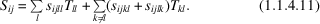The sums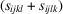for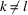have a definite physical meaning, but it is impossible to devise an experiment permittingand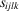to be measured separately. It is therefore usual to set them equal in order to avoid an unnecessary constant: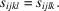Furthermore, the left-hand term of (1.1.4.11)remains unchanged if we interchange the indices i and j. The terms on the right-hand side therefore also remain unchanged, whatever the value of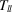or. It follows that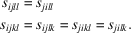Similar relations hold for,,and: the submatrices 2 and 3, 4 and 7, 5, 6, 8 and 9, respectively, are equal.

Equation (1.4.1.11)can be rewritten, introducing the coefficients of the Voigt strain matrix: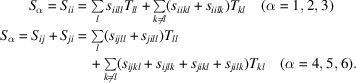We shall now introduce a two-index notation for the elastic compliances, according to the following conventions: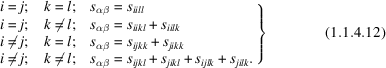We have thus associated with the fourth-rank tensor a square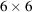matrix with 36 coefficients:One can translate relation (1.1.4.12)using thematrix representingby adding term by term the coefficients of submatrices 2 and 3, 4 and 7 and 5, 6, 8 and 9, respectively:Using the two-index notation, equation (1.1.4.9)becomes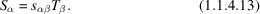A similar development can be applied to the other fourth-rank tensors, which will be replaced bymatrices with 36 coefficients, according to the following rules.

 (i) Elastic stiffnesses,and elasto-optic coefficients,:where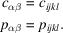(ii) Piezo-optic coefficients,:where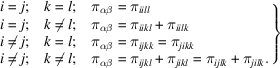(iii) Electrostriction coefficients,: same relation as for the elastic compliances.